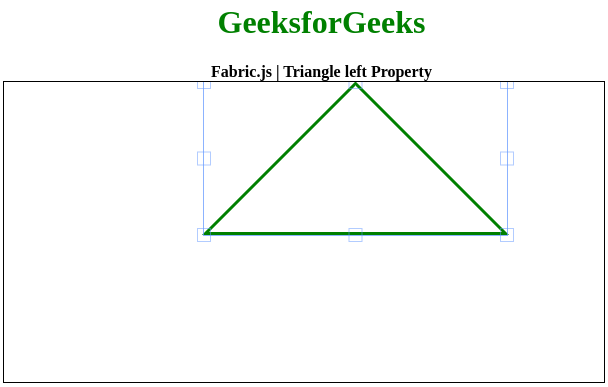Related Articles

# Fabric.js | Triangle left Property

• Last Updated : 27 May, 2020

In this article, we are going to see how to set the position relative to the left of the canvas triangle using FabricJS. The canvas triangle means the triangle is movable and can be stretched according to requirement. Further, the triangle can be customized when it comes to initial stroke color, height, width, fill color, or stroke width.

To make it possible we are going to use a JavaScript library called FabricJS. After importing the library, we will create a canvas block in the body tag that will contain the triangle. After this, we will initialize instances of Canvas and Triangle provided by FabricJS and set the position relative to left of the canvas triangle using left property and render the Triangle on the Canvas as given in the below example.

Syntax:

```fabric.Triangle({
width: number,
height: number,
left: number
});```

Parameters: This function accepts three parameters as mentioned above and described below:

• width: It specifies the width of triangle.
• height: It specifies the height of triangle.
• left: It specifies the distance from left end of canvas.

Example: This example uses FabricJS to set the position relative to the left of the canvas triangle as given below.

 ` `` `` ` ` ``    `` ``        ``Fabric.js | Triangle left Property``    `` ``     ` `    ````    `` `` `` ` ` ``    ``
``      ``

``       ``GeeksforGeeks``      ``

``      ````       ``Fabric.js | Triangle left Property``      ````    ``
``    `` ``    `` ``     ` `    `` `` `` ` ``

Output:My Personal Notes arrow_drop_up